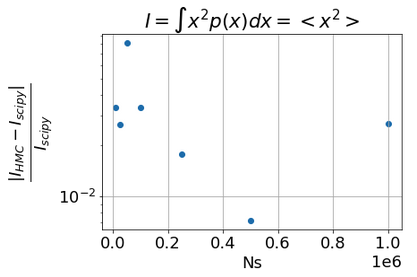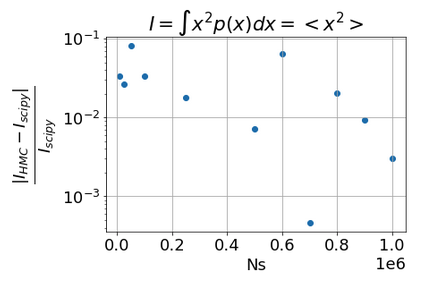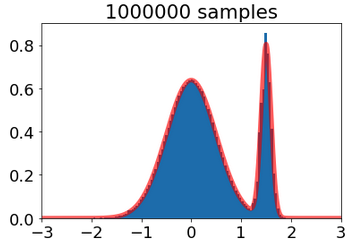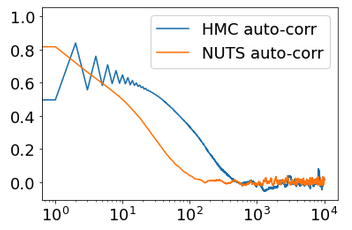# Running several chains does not improve accuracy: do you see why?

Dear Experts
Below you will find 4 questions (Q1 to Q4) and hope that the code example is running for you for investigation. Of course your comments are welcome.

import scipy.integrate as integrate
import numpy as np

import jax
import jax.numpy as jnp
from jax import grad, jit, vmap
from jax import jacfwd, jacrev, hessian
from jax.ops import index, index_update

import numpyro
from numpyro.infer import NUTS, HMC, MCMC

class MixtureModel_jax():
def __init__(self, locs, scales, weights, *args, **kwargs):
super().__init__(*args, **kwargs)
self.loc = jnp.array([locs]).T
self.scale = jnp.array([scales]).T
self.weights = jnp.array([weights]).T
norm = jnp.sum(self.weights)
self.weights = self.weights/norm

self.num_distr = len(locs)

def pdf(self, x):
probs = jax.scipy.stats.norm.pdf(x,loc=self.loc, scale=self.scale)
return jnp.dot(self.weights.T,probs).squeeze()

def logpdf(self, x):
log_probs = jax.scipy.stats.norm.logpdf(x,loc=self.loc, scale=self.scale)
return jax.scipy.special.logsumexp(jnp.log(self.weights) + log_probs, axis=0)

mixture_gaussian_model = MixtureModel_jax([0,1.5],[0.5,0.1],[8,2])

def phi(x):
return x**2

rng_key = jax.random.PRNGKey(0)
_, rng_key = jax.random.split(rng_key)

kernel = HMC(potential_fn=lambda x: -mixture_gaussian_model.logpdf(x))  # negative log
num_samples = 100_000
n_chains    = 1
mcmc = MCMC(kernel, num_warmup=2_000, num_samples=num_samples, num_chains=n_chains)
mcmc.run(rng_key, init_params=jnp.zeros(n_chains))
samples_1 = mcmc.get_samples()


Then,

phi_spl1 = phi(samples_1)
Integ_HMC1 = np.mean(phi_spl1)
print(f"Integ_HMC:{Integ_HMC1:.6e}, err. relat: {np.abs(Integ_HMC1-Integ_true)/Integ_true:.6e}")


one gets

Integ_HMC:6.521168e-01, err. relat: 1.792710e-04


Now, If I run the same code with

num_samples = 100_000
n_chains    = 10


I get,

Integ_HMC:6.608779e-01, err. relat: 1.361655e-02


Q1: Why using 10 chains is worse then a single chain while I was expecting a 1/sqrt(10) better accuracy?

Now, using NUTS in place of HMC, with

num_samples = 100_000
n_chains    = 1


I get

Integ_NUTS:6.545689e-01, err. relat: 3.940032e-03


and with

num_samples = 100_000
n_chains    = 1


the result is

Integ_NUTS:6.580432e-01, err. relat: 9.268797e-03


which shows also that running 10x more chains does not help at all.

Q2: why NUTS not better than HMC?

Q3: As a side effect: the results were obtained on a CPU and I face the problem that running a single chain of 10^6 samples would get 30mins or so, while 10 chains of 10^5 samples each is done in 30sec?

Q4: running on a single GPU (K80) or even 4GPUs does not improve the running speed. Do you catch a reason for that?

PS: I have create an issue on Pyro github but I am not sure that it is the correct way. Hope that you can close the issue in case it was not the right way to ask the question.

Hi @campagne, we recommend using the forum for questions cause it will be more visible to other users.

Quoted from the github issue:

I think multi-chain is useful for diagnosing if mcmc run is converging. As long as things converge, it is enough to use 1 chain, unless you want some speed-up (if any) from multiple chains.

why NUTS not better than HMC?

If the trajectory length is good, no need to use NUTS.

running a single chain of 10^6 samples would get 30mins or so, while 10 chains of 10^5 samples each is done in 30sec

Interesting. Probably the cost to use a placeholder of size (10^6 x latent_size) and update the value at a particular index at each step is dominating the inference cost. This might happen when latent_size is large.

For a large number of samples, you might want to disable the progress bar by setting progress_bar=False. It will hugely speed up your sampling.

running on a single GPU (K80) or even 4GPUs does not improve the running speed

Sequential methods like MCMC would be slow on GPU.

What a quick return! Thanks @fehiepsi. Now,

1. what about my original Q1 which asking why the 1/sqrt(Nsamples) does not seem to fit? It is even worse when I generate 10^7 samples as the accuracy of 10^5 sample does not improve at all. To be more specific here is a plot of relative error on <x^2> where x is supposed to be sampled by HMCand after completing by new Ns values above 500_000This is quite strange because the distribution of the samples seems quite okI have x-cheked that this is not a rounding error as np.sum and math.fsum give very close results for 10^6 samples (653976.8 and 653976.9).

1. concerning progress_bar=False: is there a way to get some print from time to time to see if the job is not trapped in a endless loop?
2. concerning Q3: you mention a placeholder of size 10^6 x latent_size: do you mean that the total samples are processed at once ??? ie not one by one?
3. concerning multi-chains on CPU: I am running on multi-cores CPU so why the chains cannot be processed in parallel as stated by the warning message
UserWarning: There are not enough devices to run parallel chains: expected 10 but got 1.


Thanks

Hi @campagne, I’m not sure if I understand Q1. You might try different seeds,… to see if it is related.

is there a way to get some print from time to time to see if the job is not trapped in a endless loop

Currently, we didn’t support it because it might affect the performance. You might want to run with progress_bar=True first to see if things work. Or use a small number of samples.

do you mean that the total samples are processed at once ??? ie not one by one?

It is just an empty array X with size 1e6 x latent_size at the start. At step i, X[i] will take the current mcmc value.

the warning message

I think the warning message will tell you that you need to set host_device_count?

Ok Thanks @fehiepsi. For the Q1, it is a rather simple result:

I = \int x^2 p(x)dx \approx \sum_{i=1}^N x_i^2


where xi are generated according to p(x) density probability. And the accuracy on the mean scales as 1/sqrt(N). So it is why I am rather surprised on the results I get when generating xi with HMC. it is like HMC samples > 500,000 are correlated ?

Could you check if it is? I just think this is a random seed issue. With some seed, you get good accuracy, with some seed, you get worse accuracy. I guess the accuracy ~ scale that you got is based on the assumption that all samples are independently drawn from the posterior. That might not be the case here…

You can use mcmc.print_summary() to see how many efficient samples that you have.Edit: I just run the code and found that with progress_bar=False your code just took a few seconds to get 1e6 samples. NUTS gives much better number of effective samples than HMC. This is a multimodal posterior so samples will likely to be correlated. You can also set MCMC(..., thinning=10) to remedy the correlation issue. In my test with NUTS, I got 20k effective samples per the total 100k samples.

Ho ! Great @fehiepsi
So let me try to understand:

1. when I use mcmc.print_summaty below

Is it the n_eff that gives the number of effective samples? ie. ~ 551 are independently generated when I was expecting 100_000 ???

1. Is it correct that if you set thinning=10then you select “1 sample over 10” after the warm up, so in the above example , I would get 10_000 samples ?

PS: I have run HMC and NUTS with both 100_000 samples (2_000 warmup) and no thinning. Then I’ve computed the auto-correlation of the samples wrt the lag: here is the result:If I am correct the NUTS curve shows that the decorrelation occurs at lag=10^2 while it is 10^3 for HMC. Ok, that sounds like NUTS gives more independent samples as 1 sample is rather correlated to its 100 followers, while for HMC the sample is correlated to its 1000 followers. If I am right then does the thinning would be set to 100 for NUTS and 1000 for HMC ? But it may be a bad solution to use thinning…

In another exercice (fit a simple polynomial model over data with noise), using NUTS with 2000 samples, I get n_eff values depening on the parameters output but more surprisingly one of the n_eff is arround 25_000 so more than 10x bigger that the samples I have set???

Yes

551 are independently generated when I was expecting 100_000 ???

I’m not sure. I would interpret it in that sense. Stan has a great article for it.

Is it correct that if you set thinning=10 then you select “1 sample over 10” after the warm up

Yes. I guess the doc for thinning is not clear enough. Could you create a PR to improve it?

If I am right then does the thinning would be set to 100 for NUTS and 1000 for HMC ?

It depends. Thinning is a typical technique to deal with correlated mcmc samples. HMC might not be a good sampler for multimodal models.

n_eff is arround 25_000 so more than 10x bigger that the samples I have set???

It might happen. You might find some information in the above Stan article. (also [this stackexchange thread might be related)

Thanks @fehiepsi

Does the n_efffactor is computed for the sample of a single chain or the whole sample set if several chains are used for mcmc.run?

Concerning the run of several chain

mcmc = MCMC(kernel, num_warmup=2_000, num_samples=num_samples, num_chains=n_chains)
mcmc.run(rng_key, init_params=jnp.zeros(n_chains))


Does the way to make rather independent chains is to use instead of jnp.zerosa version with random variable generation ?